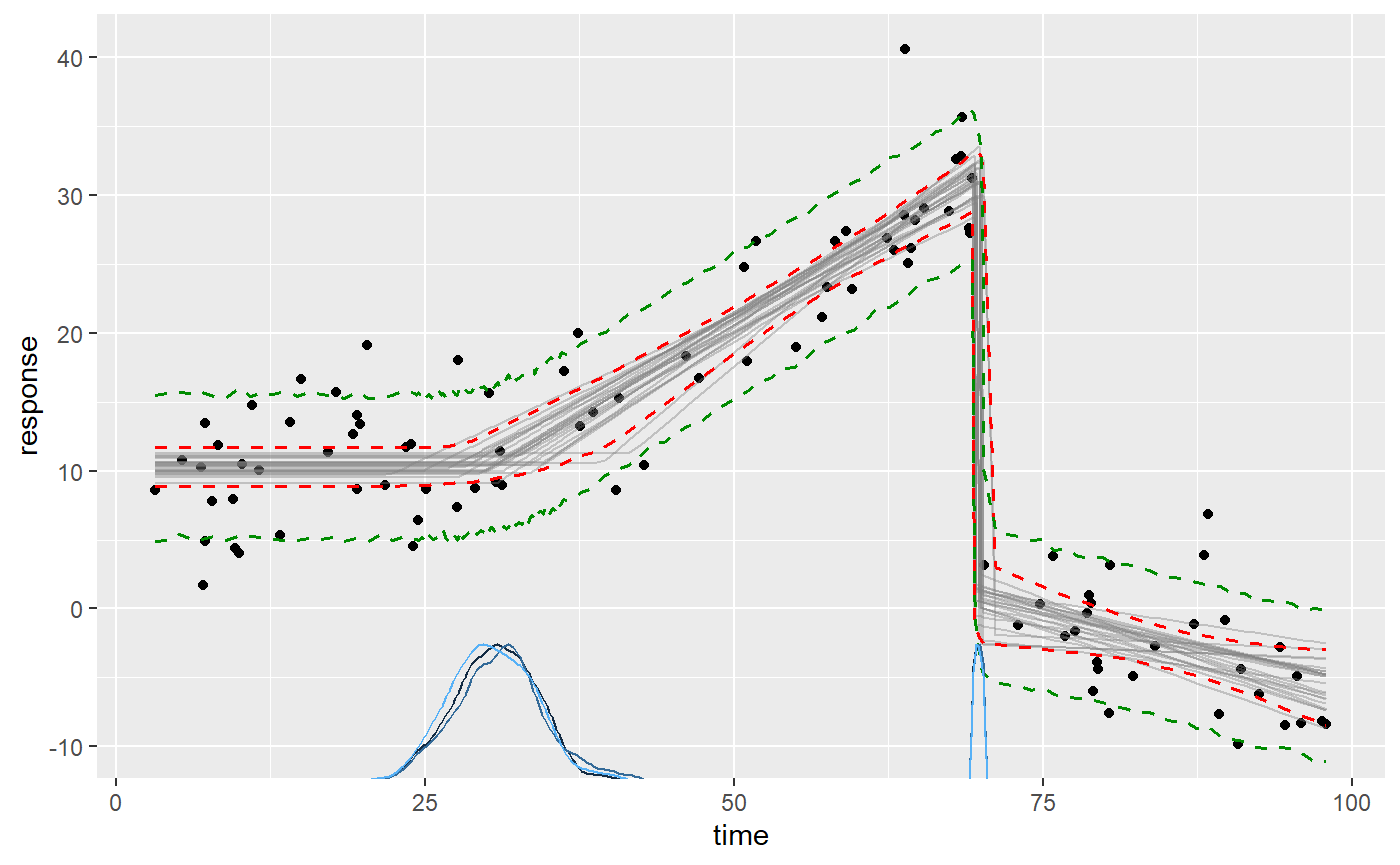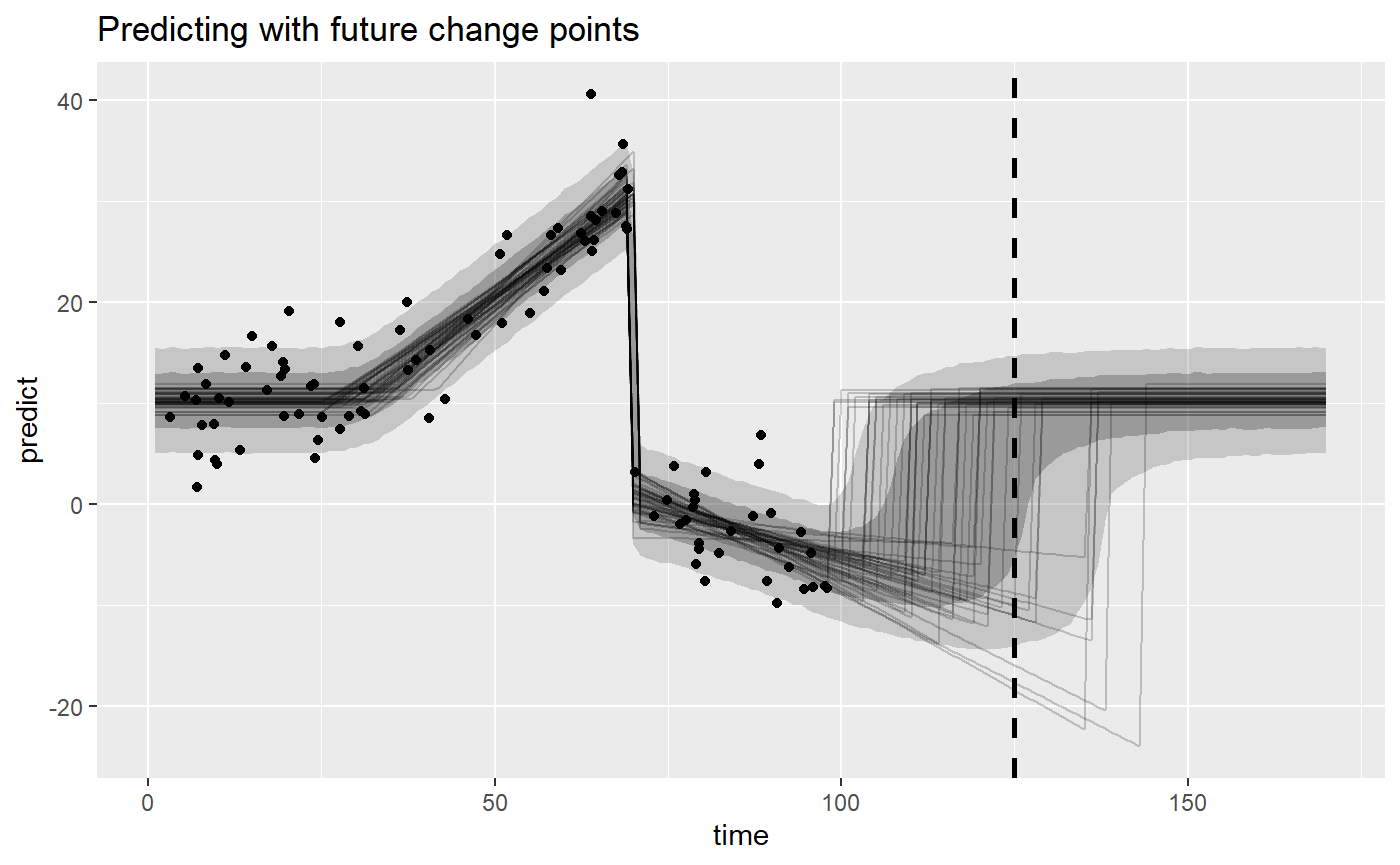This article introduces predict(), fitted(), residuals() for in-sample and out-of-sample data. I will also show how to get creative with mcp, including how to make predictions around future change points.

# Preparation: an example model

We need an mcpfit to get started. We take the “demo” dataset:

library(mcp)
options(mc.cores = 3)  # Speed up sampling
set.seed(42)  # Make the script deterministic

ex = mcp_example("demo")
head(ex$data) ## # A tibble: 6 x 2 ## time response ## <dbl> <dbl> ## 1 68.4 32.8 ## 2 87.3 -1.16 ## 3 69.0 27.6 ## 4 11.6 10.1 ## 5 19.5 14.1 ## 6 46.1 18.3 … and model it as three segments, i.e., two change points: # Define the model model = list( response ~ 1, # plateau (int_1) ~ 0 + time, # joined slope (time_2) at cp_1 ~ 1 + time # disjoined slope (int_3, time_3) at cp_2 ) # Fit it fit = mcp(model, data = ex$data)

This is what the data and the inferred fit looks like with 95% credible interval and a 80% prediction interval:

plot(fit, q_fit = TRUE, q_predict = c(0.1, 0.9))To review what we see here:

• The black dots is the data from ex$data. • The gray lines are 25 samples from the posterior (control using plot(fit, lines = 100)). • The dashed red lines are the 2.5% and 97.% quantiles of the fitted (expected) values. • The green lines are the 10% and 90% quantiles of the predicted values. • The blue curves on the x-axis are the posterior distributions of the change point locations (better viewed using plot_pars(fit, pars = c("cp_1", "cp_2"))). Behind the scenes, plot() merely calls predict() and fitted() to show these inferences. # Extracting fitted() values To get the fitted values for each data point, simply do fitted(fit): head(fitted(fit)) ## time fitted error Q2.5 Q97.5 ## 1 68.35820 30.352795 1.0524240 28.281702 32.375971 ## 2 87.29038 -3.316264 0.7639949 -4.833468 -1.826587 ## 3 69.01173 30.704731 1.0839745 28.566277 32.786091 ## 4 11.59361 10.302963 0.7323588 8.826560 11.720457 ## 5 19.50091 10.311477 0.7238347 8.878012 11.722323 ## 6 46.12009 18.377166 1.0029739 16.038041 20.057603 In general, this output will include: • A column for each predictor column in the data. Here, time is the only predictor and you see the values in the same order as in ex$data (which is copied to fit$data). But models with varying change points will additionally have a column for the varying data, binomial() models include the number of trials, etc. • fitted: The fitted value which is the mean of the posterior for this predictor value (or set of predictor values). I.e., it is the Expected Value (EV). • error:: The standard error corresponding to fitted, i.e., diff(fitted + c(-1, 1) * error) is the 68% credible interval. • Q[some number]: The quantiles of the fitted distribution. You can set the quantiles using fitted(fit, probs = c(0.1, 0.5, 0.9)). If you compare these values to the plot, you will see that they correspond. plot() merely calls fitted() behind the scenes. ## Expected values for out-of-sample data To predict out-of-sample data, you can simply use the newdata argument. newdata = data.frame(time = c(ex$data$time, 25, -20, 200)) fitted(fit, newdata = newdata) ## time fitted error Q2.5 Q97.5 ## 1 68.3582 30.35279 1.0524240 28.281702 32.375971 ## 2 25.0000 10.34998 0.7175872 8.967093 11.770927 ## 3 -20.0000 10.30119 0.7382389 8.825298 11.720457 ## 4 200.0000 -28.14998 10.6442779 -49.429380 -7.991097 Note that: • We get one row per value of time. • The first value for time is in the dataset. The values correspond to the same row in fitted(fit) because that’s merely a shortcut to do fitted(fit, newdata = fit$data).
• The second value (time = 20) is within the observed region, but not in the dataset.
• The third value (time = - 20) is outside the observed region, but mcp merely extends the first segment backwards in time. Because it’s a plateau, we see approximately the same values as for time = 20.
• The fourth value (time = 200) is way outside the observed region. Because it is the extrapolation of the slope in the third segment of which we’ve only observed the first tiny bit, the posterior distribution is very wide because even a small uncertainty in the slope results in very large differences further out.

## Arguments

If you look at the documentation for predict(), fitted(), and residuals(), you’ll see that they are quite versatile, taking many different arguments. To mention a few, you can set which_y = "sigma" to get fitted values for sigma more on modeling sigma, prior = TRUE to predict using only the prior, varying = TRUE and arma = FALSE can be toggled to include/exclude AR(N) and varying effects.

# Predictions

predict() is the posterior predictive and it takes exactly the same arguments as fitted(). This means that you can make predictions for in-sample and out-of-sample data as well. As with fitted(), plot() uses predict() under the hood to plot prediction intervals. You can see that the values correspond to dashed green lines in the plot (the 80% prediction interval):

set.seed(42)
head(predict(fit, probs = c(0.1, 0.9)))
##       time   predict    error       Q10       Q90
## 1 68.35820 30.275914 4.184053 24.972560 35.608088
## 2 87.29038 -3.339362 4.101321 -8.619435  1.880431
## 3 69.01173 30.764242 4.148573 25.449155 36.020085
## 4 11.59361 10.269686 4.077599  5.100657 15.494820
## 5 19.50091 10.195011 4.107429  4.956546 15.454846
## 6 46.12009 18.388889 4.124531 13.147433 23.702492

Note that predict() uses random sampling under the hood, so these values will differ slightly from call to call. You can make it replicable using set.seed() as above. In general, the more posterior samples, the less the call-to-call variance will be. Conversely, fewer samples means more call-to-call variation, e.g., if you do predict(fit, nsamples = 10)).

# Residuals

## Step 2: add the unobserved segment(s) and fit

Now we extend the model with the future segment of which we have prior knowledge:

model_forecast = c(fit$model, list( ~ 1 # intercept (int_4) after cp_3 )) And finally, we extend the list of priors with the two new parameters (time_4 and cp_3). It may be helpful to review the article on priors in mcp. prior_forecast = c(fit$prior, list(
cp_3 = "dnorm(cp_2 + (cp_2 - cp_1), 20) T(MAXX, )"  # In the future at the same interval
))

Now let’s fit it:

fit_forecast = mcp(model_forecast, data = ex$data, prior = prior_forecast) ## Step 3: predict! We can go right ahead and compute our 50% and 80% prediction intervals at time = 125: predict(fit_forecast, newdata = data.frame(time = 125), probs = c(0.1, 0.25, 0.75, 0.9)) ## time predict error Q10 Q25 Q75 Q90 ## 1 125 3.946098 10.99492 -13.8512 -5.578755 11.79398 14.61287 To really understand what’s going on here, it may be helpful to visualize the model. For now, we will have to hack this a bit too, manually doing our plot: # Get posterior and posterior predictive "predictions" newdata = data.frame(time = 1:170) fitted_forecast = fitted(fit_forecast, newdata = newdata, summary = FALSE, nsamples = 50) predict_forecast = predict(fit_forecast, newdata = newdata, summary = FALSE) # Plot it library(ggplot2) ggplot(predict_forecast, aes(x = time, y = predict)) + # Prediction intervals and line at x = 125 stat_summary(fun.data = median_hilow, fun.args = list(conf.int = 0.8), geom = "ribbon", alpha = 0.2) + stat_summary(fun.data = median_hilow, fun.args = list(conf.int = 0.5), geom = "ribbon", alpha = 0.3) + geom_vline(xintercept = 125, lty = 2, lwd = 1) + # Lines for fitted draws geom_line(aes(y = fitted, group = .draw), data = fitted_forecast, alpha = 0.2) + # Observed data geom_point(aes(x = time, y = response), data = ex$data) +
labs(title = "Predicting with future change points")You can read the predicted values from above at x = 125 off this graph. We literally just predicted for all values between 1 and 170, and visualized it using a ribbon. This means that you can also predict further into the future, if you’d like.

You can extend this approach to an arbitrary number of future segments, even using the posterior from the “unobserved” segment 4 in the priors for parameters in future segments. In Bayesian inference, it really does not make much of a difference whether credence in some parameter values have been updated using data or not - it’s all credence.

Without doing this formal model of the future change point, one may have thought that the change point should occur around time = 110 since that’s the expected value of cp_2 + (cp_2 - cp_1). However, we truncated the prior for the future change point (cp_3) so that it occurs after the last data point (MAXX), i.e., at time > 100. This is knowledge that the third change point had not yet been observed at time = 100, and this pushes the distribution further into the future (actually around 118; see summary(fit_forecast)).